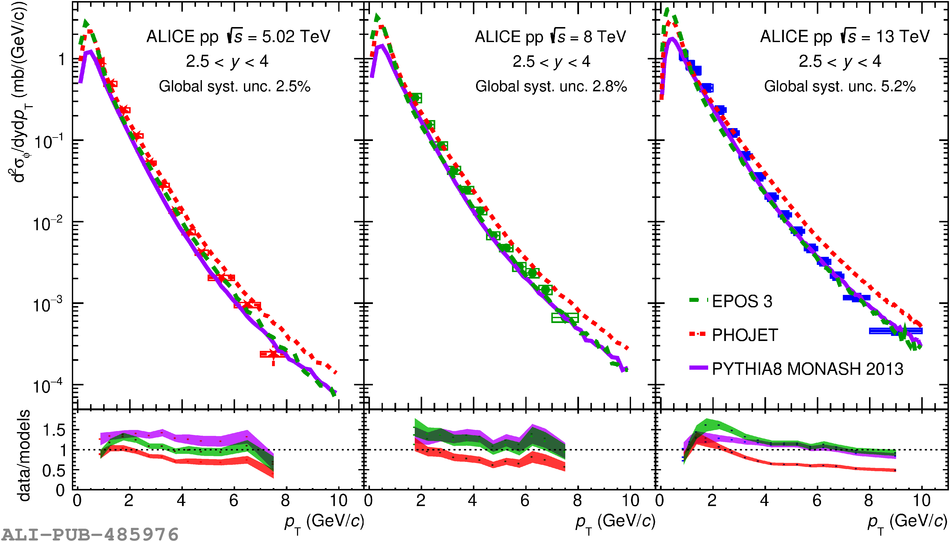Figure 4

 Top: differential $\phi$ meson production cross section $\mathrm{d}^2\sigma/\mathrm{d}y\mathrm{d}\pt$ as a function of $\pt$ at $\sqrt{s}=5.02$~TeV (left), $\sqrt{s}=8$~TeV (center) and $\sqrt{s}=13$~TeV (right) in the rapidity interval \$2.5< y compared="" with="" epos="" phojet="" and="" the="" monash="" tune="" of="" pythia="" boxes="" represent="" systematic="" uncertainties="" error="" bars="" statistical="" bottom:="" ratio="" between="" measured="" cross="" section="" calculations.="">< /y>